• 滤除缺失数据dropna()1)滤除含有NaN值的所有2)滤除含有NaN值的所有列3)滤除元素都是NaN值的4)滤除元素都是NaN值的列5)滤除指定列中含有缺失的2.删除重复值 drop_duplicates()3.根据指定条件删除行列drop() 1....

1.滤除缺失数据dropna()

import pandas as pd
import numpy as np
df=pd.DataFrame({"record":[np.nan,"亚健康|潘光|45岁","疾病|张思",np.nan],"date":[np.nan,20210102,20210103,20210104]},index=["one","two","three","four"])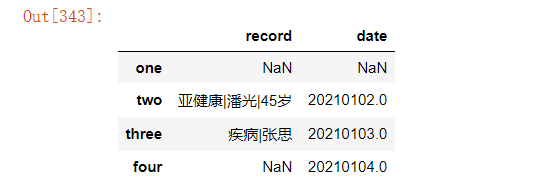1)滤除含有NaN值的所有行

df.dropna()#默认axis=0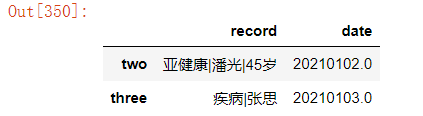2)滤除含有NaN值的所有列

df.dropna(axis=1)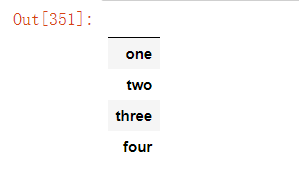3)滤除元素都是NaN值的行

df.dropna(axis=0,how="all")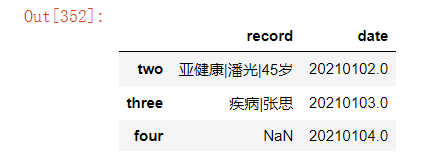4)滤除元素都是NaN值的列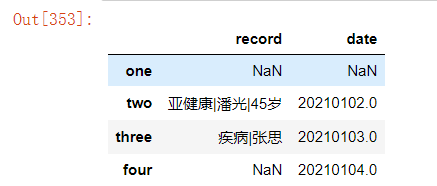5)滤除指定列中含有缺失的行

df.dropna(subset=["record"],axis=0)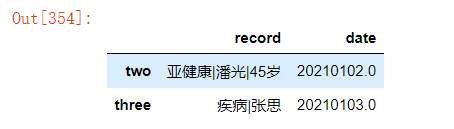以上如果需要在原数据上直接做更改，需设置参数inplace=True

2.删除重复值 drop_duplicates()

df=pd.DataFrame({'state':[1,1,2,2,1,2,2],'pop':['a','b','c','d','b','c','d']})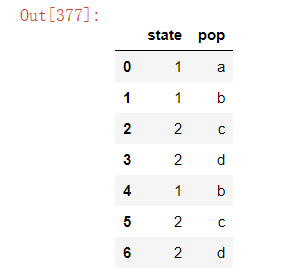语法：drop_duplicates(subset,keep,inplace)，其中参数 keep:{‘first’，‘last’，False}，默认’first’

• first：保留第一次出现的重复项，删除第二次及之后出现的重复项。

• last：保留最后一次出现的重复项，删除之前出现的重复项。

• "false"：删除所有重复项。

1)keep=“first”

df.drop_duplicates(keep="first")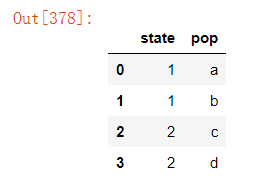2)keep=“last”

df.drop_duplicates(keep="last")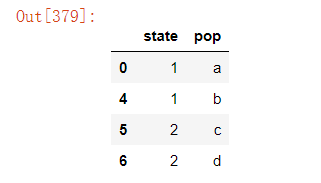3)keep=False

df.drop_duplicates(keep=False)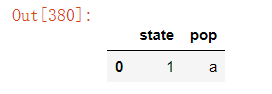4)删除指定列中重复项对应的行

df.drop_duplicates(subset=["state"],keep="first")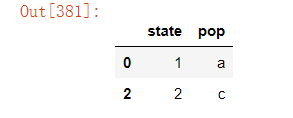以上如果需要在原数据上直接做更改，需设置参数inplace=True

3.根据指定条件删除行列drop()

df=pd.DataFrame(np.arange(16).reshape(4,4),columns=["one","two","three","four"])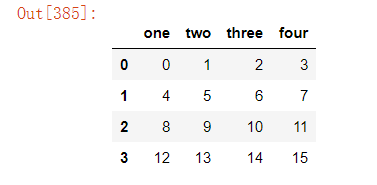1).删除指定列

df.drop(["one"],axis=1)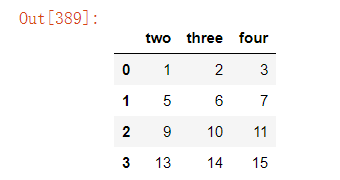另外，也可通过del df["one"]来实现删除指定列，但该方法不推荐，因为这默认直接在源数据上做更改。

2).删除指定行

df.drop(,axis=0)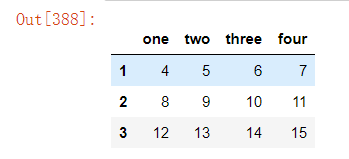以上如果需要在原数据上直接做更改，需设置参数inplace=True

展开全文python pandas
• pandas删除指定行详解

千次阅读 2020-12-19 14:37:33
pandas删除指定行详解在处理pandas的DataFrame中，如果想像excel那样筛选，只要其中的某一行或者几行，可以使用isin()方法来实现，只需要将需要的行值以列表方式传入即可，还可传入字典，进行指定筛选。pandas....

pandas删除指定行详解

在处理pandas的DataFrame中，如果想像excel那样筛选，只要其中的某一行或者几行，可以使用isin()方法来实现，只需要将需要的行值以列表方式传入即可，还可传入字典，进行指定筛选。pandas.DataFrame中删除包涵特定字符串所在的行：https://www.jb51.net/article/159052.htm

以上所述是小编给大家介绍的pandas删除指定行详解整合，希望对大家有所帮助，如果大家有任何疑问请给我留言，小编会及时回复大家的。在此也非常感谢大家对我们网站的支持！

时间： 2019-04-01

用法:DataFrame.drop(labels=None,axis=0, index=None, columns=None, inplace=False) 在这里默认:axis=0,指删除index,因此删除columns时要指定axis=1: inplace=False,默认该删除操作不改变原数据,而是返回一个执行删除操作后的新dataframe: inplace=True,则会直接在原数据上进行删除操作,删除后就回不来了. 例子: >>>df = pd.DataFrame(np.a

假如有一列全是字符串的dataframe,希望提取包含特定字符的所有数据,该如何提取呢? 因为之前尝试使用filter,发现行不通,最终找到这个行得通的方法. 举例说明: 我希望提取所有包含'Mr.'的人名 1.首先将他们进行字符串化,并得到其对应的布尔值: >>> bool = df.s

展开全文• 目标：删除第四列值为0的全部数据 原本的数据集 代码： d=[] for i, row in data.iterrows(): if row == 0: d.append(i) data=data.drop(d) data.shape DataFrame.iterrows(self)：将DataFrame...

目标：删除第四列值为0的全部数据

原本的数据集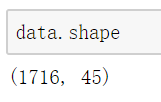代码：

d=[]
for i, row in data.iterrows():
if row == 0:
d.append(i)

data=data.drop(d)
data.shape

DataFrame.iterrows(self)：将DataFrame行作为（索引，Series）对进行迭代。

按行遍历，i表示行的索引，row表示那一行的数据，row就是第i行的第4列的数据，若它为0，则将那一行的索引放入列表中，再从数据集中删除不符合要求的数据。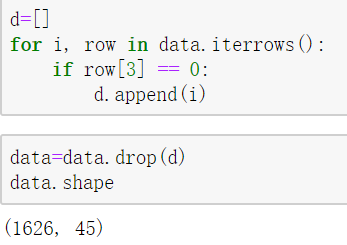展开全文• 使用df.drop DataFrame.drop(labels=...2、axis：指定哪一个轴，=0删除行，=1删除列； 3、columns：指定某一列或者多列(以list形式)； 4、level：索引等级，针对多重索引的情况； 5、inplaces：是否替换原来的datafram

使用df.drop

DataFrame.drop(labels=None, axis=0, index=None, columns=None, level=None, inplace=False, errors=‘raise’)
1、labels：要删除的标签，一个或者多个(以list形式)；
2、axis：指定哪一个轴，=0删除行，=1删除列；
3、columns：指定某一列或者多列(以list形式)；
4、level：索引等级，针对多重索引的情况；
5、inplaces：是否替换原来的dataframe，=True代表直接替换原始df，=False代表不替换原始df

1、直接删除指定行/列

# 删除列名为C的列，并替换原始df
df.drop(columns=['C'], inplace=True)

2、根据条件删除指定行

# 删除符合条件的指定行，并替换原始df
df.drop(df[(df.score < 50) & (df.score > 20)].index， inplace=True)

展开全文• df.dropna(axis=0) #删除有空值的，使用参数axis=0 df.dropna(axis=1) #删除有空值的列，使用参数axis=1 drop解释 Ipython:dataframe.drop? Signature: dataframe.drop( labels=None, ...pandas drop 删除空行
• 我想删除一些并将其余数据转换为浮点数.我想删除’X’或’XX’的,其余的我应该转换为float,数字像4; 00.1应该转换为4.001该文件看起来像这样的样本：0,1,10/09/2012,3:01,4;09.1,5,6,7,8,9,10,111,-0.581586,11/...
• import pandas as pd df = pd.read_csv('./1111.csv') print('原来的是: ', df.shape) y = df[df['filename'].str.contains('other')] # 这里填写需要删除的关键字！！！！！！！！！ print('需要删除: ', y.shape...python
• pandas 删除某一/列 方法1 # pandas 删除某一/列 # 语法：DataFrame.drop(labels=None,axis=0, index=None, columns=None, inplace=False) 参数说明： labels 就是要删除的行列的名字，用列表给定 axis 默认为...python 开发语言 后端
•python
• 一次性删除指定多列均为空的数据 df_last.dropna(subset=['amdlevel','focuspath','imgcoord'],inplace=True)
• df _= df[~df.id.isin(['a','b','c'])] 结果为df保留除了‘a’,‘b’,‘c’的三数据python pandas
• 我想要一个简单的方法来删除数据帧内指定字符后的字符串部分.这是一个简化的例子：DF：obs a b c d0 1 1-23-12 1 2 31 2 12-23-13 4 5 52 3 21-23-14 4 5 5我想在第一个符号后删除a列中的部分,我的预期输出是：newdf...
• 现有数据两列，如下图：然后我想删除a列中符合某正则表达式(如0002开头)的数据。该如何写？添加：前面只是举例哈，因为不一定是XXXX开头，所以更希望是用re.正则表达式去匹配。谢谢楼下的回答。另：现在遇到另个小...
• df.dropna(subset = [‘需要考虑空值的列’])pandas
• pandas初步删除明显错误的值，对数据做初步清洗 def del_error_data(self, data): data = data[data.IAS.between(0, 70, inclusive=True)] data = data[data.Throttle.between(0, 115, inclusive=True)] data = ...python
• dataframe、series如何删除指定列中有空值的+如何删除多列都为空的pandas
• pandas删除空值

2021-08-22 22:42:52
pandas 删除空值 目的： 将该含有过多空值的/列删除 DataFrame.dropna(axis=0, how='any', thresh=None, subset=None, inplace=False) 参数解释： axis:维度，axis=0表示index,axis=1表示columns列，默认为0 ...
• 小编典典 这个问题已经解决，但是… …还要考虑伍特（Wouter）在其原始评论中提出的解决方案。dropna()大熊猫内置了处理丢失数据（包括）的功能。除了通过手动执行可能...上的文档），包括删除列而不是。 2020-02-15
• In : df = pd.DataFrame(dict(A=[5,3,5,6], C=[“foo”,“bar”,“fooXYZbar”, “bat”])) In : df Out: A C 0 5 foo 1 3 bar 2 5 fooXYZbar 3 6 bat In : df=df[~df.C.str.contains(“XYZ”)] ...机器学习
• 开始之前，pandas中DataFrame删除对象可能存在几种情况 1、删除具体列 2、删除具体 3、删除包含某些数值的或者列 4、删除包含某些字符、文字的或者列 本文就针对这四种情况探讨一下如何操作。 数据准备 模拟了...python pandas
• #保留A，B，C列的NAN，删除其他所有NAN df = df[df[‘A’ or ‘B’ or ‘C’].notnull()]python
• pandas 的drop（）不能删除nan值的python 机器学习 数据挖掘
• 用法：DataFrame.drop(labels=None,axis=0, index=None, columns=None, inplace=False)在这里默认：axis=0，指删除index，因此删除columns时要指定axis=1；inplace=False，默认该删除操作不改变原数据，而是返回一个...
• python--pandas删除操作

千次阅读 2021-04-12 17:26:27
删除是数据清洗中的高频操作，本文基于pandas，介绍其dataFrame的一些删除操作，包括了删除行删除列，删除缺失值，删除重复值。 环境 python3.9 win10 64bit pandas==1.2.1 本文介绍的方法中，均有inplace...python 数据分析
• 您可以应用一个功能,以方式测试您的DataFrame是否存在字符串,例如,说df是您的DataFramerows_with_strings = df.apply(lambda row :any([ isinstance(e, basestring) for e in row ]), axis=1)这将为您的DataFrame...
• 1.按列取、按索引/取、按特定行列取 import numpy as np from pandas import DataFrame import pandas as pd df=DataFrame(np.arange(12).reshape((3,4)),index=[‘one’,‘two’,‘thr’],columns=list(‘abcd’)...
• 下面是我拥有的pandas数据框的子集,我试图根据某些条件删除多行.code1 code2 grp1 grp2 dist_km0 M001 M002 AAA AAA 1121 M001 M003 AAA IHH 2752 M002 M005 AAA XXY 1503 M002 M004 AAA AAA 654 M003 ...
• 1.删除/选取某列含有特殊数值的import pandas as pdimport numpy as npa=np.array([[1,2,3],[4,5,6],[7,8,9]])df1=pd.DataFrame(a,index=['row0','row1','row2'],columns=list('ABC'))print(df1)df2=df1.copy()#......